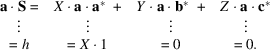International
Tables for
Crystallography
Volume F
Crystallography of biological macromolecules
Edited by E. Arnold, D. M. Himmel and M. G. Rossmann

International Tables for Crystallography (2012). Vol. F, ch. 2.1, pp. 57-58   | 1 | 2 |

## Section 2.1.5. Reciprocal space and the Ewald sphere

J. Drentha*

aLaboratory of Biophysical Chemistry, University of Groningen, Nijenborgh 4, 9747 AG Groningen, The Netherlands
Correspondence e-mail: j.drenth@chem.rug.nl

### 2.1.5. Reciprocal space and the Ewald sphere

| top | pdf |

A most convenient tool in X-ray crystallography is the reciprocal lattice. Unlike real or direct space, reciprocal space is imaginary. The reciprocal lattice is a superior instrument for constructing the X-ray diffraction pattern, and it will be introduced in the following way. Remember that vector S(hkl) is perpendicular to a reflecting plane and has a length(Section 2.1.4.5). This will now be applied to the boundary planes of the unit cell: the bc plane or (100), the ac plane or (010) and the ab plane or (001).

 For the bc plane or (100): indices,and; S(100) is normal to this plane and has a length. Vector S(100) will be called. For the ac plane or (010): indices,and; S(010) is normal to this plane and has a length. Vector S(010) will be called. For the ab plane or (001): indices,and; S(001) is normal to this plane and has a length. Vector S(001) will be called.

From the definition of,andand the Laue conditions [equation (2.1.4.7)],the following properties of the vectors,andcan be derived:However,andbecauseis perpendicular to the (100) plane, which contains the b and c axes. Correspondingly,and.

Proposition. The endpoints of the vectors S(hkl) form the points of a lattice constructed with the unit vectors,and.

Proof. Vector S can be split into its coordinates along the three directions,and:Our proposition is true if X, Y and Z are whole numbers and indeed they are. Multiply equation (2.1.5.1)on the left and right side by a.The conclusion is that,and, and, therefore,The diffraction by a crystal [equation (2.1.4.6)]is only different from zero if the Laue conditions [equation (2.1.4.7)]are satisfied. All vectors S(hkl) are vectors in reciprocal space ending in reciprocal-lattice points and not in between. Each vector S(hkl) is normal to the set of planes () in real space and has a length(Fig. 2.1.5.1).Figure 2.1.5.1 | top | pdf |A two-dimensional real unit cell is drawn together with its reciprocal unit cell. The reciprocal-lattice points are the endpoints of the vectors S(hk) [in three dimensions S(hkl)]; for instance, vector S(11) starts at O and ends at reciprocal-lattice point (11). Reproduced with permission from Drenth (1999). Copyright (1999) Springer-Verlag.

The reciprocal-lattice concept is most useful in constructing the directions of diffraction. The procedure is as follows:

• Step 1: Draw the vectorindicating the direction of the inci­dent beam from a point M to the origin, O, of the reciprocal lattice. As in Section 2.1.4.2, the length ofand thus the distance MO is(Fig. 2.1.5.2).Figure 2.1.5.2 | top | pdf |The circle is, in fact, a sphere with radius.indicates the direction of the incident beam and has a length. The diffracted beam is indicated by vector s, which also has a length. Only reciprocal-lattice points on the surface of the sphere are in a reflecting position. Reproduced with permission from Drenth (1999). Copyright (1999) Springer-Verlag.
• Step 2: Construct a sphere with radiusand centre M. The sphere is called the Ewald sphere. The scattering object is thought to be placed at M.

• Step 3: Move a reciprocal-lattice point P to the surface of the sphere. Reflection occurs withas the reflected beam, but only if the reciprocal-lattice point P is on the surface of the sphere, because only then does(Section 2.1.4.2). Noncrystalline objects scatter differently. Their scattered waves are not restricted to reciprocal-lattice points passing through the Ewald sphere. They scatter in all directions.